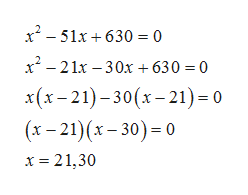(square root of x -5) plus x equals 25

Question

(square root of x -5) plus x equals 25

Step 1

Given equation is

Step 2

On squaring both sides, we get

Step 3

Now, we solve the quadratic equation by spl...help_outlineImage Transcriptionclosex2-51x630 0 x-21x 30x + 630 = 0 x(x-21)-30(x-21)- 0 (x-21)(x-30) 0 x 21,30 fullscreen

Want to see the full answer?

See Solution

Want to see this answer and more?

Our solutions are written by experts, many with advanced degrees, and available 24/7

See Solution
Tagged in

Algebra